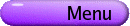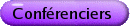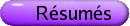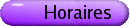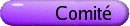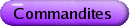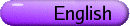Géométrie de Poisson et physique mathématique
Org: Eckhard Meinrenken (Toronto)
[PDF]

TOM BAIRD, University of Toronto, Toronto, Ontario, M5S 2E4, Canada
Moduli space of flat SU(2) bundles over nonorientable surfaces
[PDF]

I will present a computation of the cohomology groups of the moduli space of flat SU(2) bundles over a closed nonorientable surface S, which we identify via the holonomy map with X/SU(2), where X : = Hom( p1 (S), SU(2) ). The strategy will be to determine the equivariant cohomology ring H*SU(2)(X), and then pass to H* ( X/SU(2) ) via a pair of long exact sequences and localization.

OLEG BOGOYAVLENSKI, Queen's University, Kingston, Ontario, K7L 3N6
Invariant foliations with dynamical systems for the Poisson brackets of hydrodynamic type
[PDF]

An invariant foliation Fm with an induced non-degenerate metric áv,w ñ of constant curvature K is discovered for any degenerate Poisson bracket of hydrodynamic type on a manifold Mn with (2,0)-tensor gij(u) of rank m < n. An invariant dynamical system V on Mn is introduced that is tangent to the leaves of the foliation Fm. The dynamical system V is applied for constructing the scalar and tensor invariants of the Poisson bracket. Invariant (n-m)-dimensional nilpotent Lie algebras Au are found that are embedded into the cotangent spaces T*u (Mn).

## References


O. I. Bogoyavlenskij, Schouten tensor and bi-Hamiltonian systems of hydrodynamic type. J. Math. Phys. 47, 2006.


, Invariant foliations for the Poisson brackets of hydrodynamic type. Phys. Letters A (2006).

HENRIQUE BURSZTYN, IMPA
Quasi-Poisson geometry and Dirac structures
[PDF]

In this talk, I will explain how to define hamiltonian spaces with D/G-valued moment maps (where (D,G) is a group pair integrating a Manin pair) in terms of Dirac structures, and prove that this approach is equivalent to the original one of Alekseev and Kosmann-Schwarzbach based on quasi-Poisson geometry. I will explain how the two viewpoints complement one another and how they shed light on the theory of G-valued moment maps, in the sense of Alekseev, Malkin and Meinrenken.

SAM EVENS, University of Notre Dame, Notre Dame, IN 46556, USA
Poisson geometry of the Grothendieck resolution
[PDF]

We construct a Poisson structure on the Grothendieck resolution X of a complex semisimple group G. The natural map m: X ® G is Poisson with respect to a Poisson structure p on G such that closures of conjugacy classes are Poisson subvarieties. pG was first constructed by Alekseev and Malkin. We determine symplectic leaves on the Grothendieck resolution, and show that m resolves singularities of the Poisson structure p on G.

This talk is based on joint work with Jiang-Hua Lu.

SHAY FUCHS, University of Toronto, Canada
Additivity of spin-c Quantization Under Cutting
[PDF]

We describe a cutting construction for a compact oriented Riemannian manifold M, endowed with an S1-equivariant spinc structure. This produces two other equivariant spinc manifolds (the "cut spaces"), denoted by Mcut+ and Mcut-.

The spinc structures on M, Mcut+ and Mcut- (together with a connection on their determinant line bundles) enable us to define virtual representations of S1, called the "spin-c quantization" of the manifold.

We claim that the representation that corresponds to M is the sum of the representations that correspond to those of the cut spaces, and we outline the main steps in the proof.

MARCO GUALTIERI, MIT
Holomorphic Poisson D-branes
[PDF]

I will define the notion of D-brane on a holomorphic Poisson manifold and give some methods as well as consequences of their construction.

Orbifold cohomology of hypertoric varieties
[PDF]

Hypertoric varieties are hyperkähler analogues of toric varieties, and are constructed as abelian hyperkähler quotients of a quaternionic affine space. Just as symplectic toric orbifolds are determined by labelled polytopes, orbifold hypertoric varieties are intimately related to the combinatorics of hyperplane arrangements. By developing hyperkähler analogues of symplectic techniques developed by Goldin, Holm, and Knutson, we give an explicit combinatorial description of the Chen-Ruan orbifold cohomology of an orbifold hypertoric variety in terms of the combinatorial data of a rational cooriented weighted hyperplane arrangement. Time permitting, we detail several explicit examples, including some computations of orbifold Betti numbers (and Euler characteristics).

This is joint work with R. Goldin.

TARA HOLM, Cornell University, Department of Mathematics, Ithaca, NY 14853-4201 USA
Integral cohomology of symplectic quotients
[PDF]

I will describe some work in progress on computing the integral cohomology of symplectic reductions. Under a hypothesis on the isotropy groups, we may prove that the Kirwan map from equivariant cohomology of the total space to the ordinary cohomology of the reduced space, both with integer coefficients, is a surjection. We will apply this result to compute the integral cohomology of certain toric orbifolds.

This talk is based on joint work with Susan Tolman.

GREG LANDWEBER, University of Oregon, Eugene, OR 97403, USA
Equivariant formality in K-theory
[PDF]

This talk will introduce the notion of equivariant formality in K-theory. For Borel equivariant cohomology theories, equivariant formality is the statement that the Leray-Serre sequence for the fibration M ® MG ® BG collapses at the E2 stage, giving an isomorphism HG(M) @ H(M) ÄHG(pt) as modules over HG(pt). In the equivariant bundle construction of K-theory, we do not have such a fibration, so we introduce a different definition, that K(M) @ KG(M) ÄR(G) Z, tensoring down rather than tensoring up.

We will prove that compact Hamiltonian G-spaces are always equivariantly formal in K-theory, using as our main tool the Kunneth spectral sequence, and showing that the higher R(G)-torsion in KG(M) vanishes. It follows that the forgetful map KG(M) ®K(M) is surjective, and as a corollary, we will show that every complex line bundle over M admits a lift of the G-action.

This talk consists of joint work with Megumi Harada.

EUGENE LERMAN, Illinois, Urbana-Champaign
Is it useful to think of orbifolds as stacks?
[PDF]

I have been told by a number of people that one should think of orbifolds as Deligne-Mumford stacks. I have also been warned that "it is difficult to explain what stacks are and it is even more difficult to explain why it's the right way to think about orbifolds." I will report on what happened when I tried to come to grips with orbifolds as stacks.

YI LIN, University of Toronto, BA 6172, 40 St. George St., Toronto, Ontario, M5S 2E4
Hamiltonian actions on generalized complex manifolds and the equivariant [`()]-lemma
[PDF]

We first review the definition of Hamiltonian actions on generalized complex manifolds and present some non-trivial examples. Given a Hamiltonian action of a compact Lie group on a generalized complex manifold which satisfies the [`()] -lemma, we show that there is an equivariant version of the [`()]-lemma which is a direct generalization of the equivariant dG d-lemma in symplectic geometry.

JOHAN MARTENS, MPI Bonn / University of Toronto
Euler characteristics of GIT quotients
[PDF]

We will discuss work in process determining Euler characteristics over GIT quotients (symplectic reductions) as residues of expressions in equivariant K-theory. We will indicate the relationship with the Jeffrey-Kirwan theorem in cohomology.

MARKUS PFLAUM, Goethe-University, FB Mathematik, Robert-Mayer-Str. 10, 60054 Frankfurt/Main, Germany
Cyclic cohomology of deformation quantizations over orbifolds
[PDF]

In the talk, the cyclic homology of deformation quantizations of the convolution algebra over a proper etale groupoid G will be studied. It is shown that cyclic homology recovers the (additive) structure of the orbifold cohomology of the orbit space X=G0/G. As a consequence, the space of traces on the deformed convolution algebra has dimension equal to the number of sectors of the underlying orbifold. Using these results, I then elaborate on the application in the algebraic index theory of orbifolds.

Joint with N. Neumaier, H. Posthuma and X. Tang.

MARTIN PINSONNAULT, Fields Institute & University of Toronto
Maximal Tori in the Hamiltonian groups of 4-manifolds
[PDF]

Let (M,w) be a symplectic 4-manifold. Let Symp be its group of symplectomorphisms and denote by Ham its subgroup of Hamiltonian diffeomorphisms. Let M be the set of maximal tori in Ham and let T be the subset of 2-dimensional tori. Both Symp and Ham act by conjugation on M and T. We will explain why the quotient space M / Symp is finite, and describe what the finiteness of T / Ham would imply for the homotopy type of Symp.

ROMARIC PUJOL, University of Toronto
Poisson Groupoids, Duality and Classical Dynamical Yang-Baxter Equation
[PDF]

I will briefly recall basic notions about Poisson groupoids and their duality. As examples of these, I will present the class of bidynamical Poisson groupoids, and show the relations with the classical dynamical Yang-Baxter equation (CDYBE) and Lie quasi-bialgebras. From this geometric point of view, we are able to give explicit solutions of the CDYBE.

REYER SJAMAAR, Cornell University, Ithaca, New York
Torsion and abelianization in equivariant cohomology
[PDF]

Let X be a topological space upon which a compact connected Lie group G acts. It is well known that the equivariant cohomology HG* (X,Q) is isomorphic to the subalgebra of Weyl group invariants of the equivariant cohomology HT* (X,Q), where T is a maximal torus of G. We establish a similar relationship for coefficient rings more general than Q.

Our results rely on work of Grothendieck and Demazure concerning the intersection theory of flag varieties and have applications to the cohomology of homogeneous spaces and, potentially, symplectic quotients.

This is a report on joint work with Tara Holm.

XIANG TANG, Washington University, St. Louis, MO, 63130, USA
Algebraic structures on Hochschild cohomology of an orbifold
[PDF]

In this talk, we study algebraic structures on the Hochschild cohomology of the convolution algebra over a proper etale groupoid G.

We show that the Gerstenhaber bracket defines a twisted Schouten-Nijenhuis bracket between multivector fields on the corresponding inertia orbifold [^(X)] of X = G0/G. This leads an interesting connection to symplectic reflection algebra.

We will define a de Rham model for the Chen-Ruan orbifold cohomology, and explain its relations to the ring structure on the Hochschild cohomology.

Joint work with G. Halbout, N. Neumaier, M. Pflaum, H. Posthuma and H. Tseng.

Poisson fiber bundles
[PDF]

Poisson fiber bundles are natural generalizations of symplectic fiber bundles.

I will discuss an approach to Poisson fiber bundles which is based on the theory of Dirac structures on manifolds. I will review various constructions of coupling Dirac structures on manifolds.

JONATHAN WEITSMAN, Santa Cruz

GRAEME WILKIN, Johns Hopkins University
The Lojasiewicz inequality for Higgs bundles
[PDF]

An important technique in the study of symplectic (and hyperkähler) quotients is to use the Morse theory of the norm-square of the moment map. The Lojasiewicz inequality is a key estimate in the process of proving that the gradient flow of a functional converges, which is essential in order for Morse theory to work. This technique was first used for certain infinite-dimensional problems by Leon Simon, and then extended by Johan Rade to study the gradient flow of the Yang-Mills functional in two and three dimensions. Here we extend Rade's version of the Lojasiewicz inequality to functionals which are invariant under a group action and also satisfy a certain ellipticity condition on the Hessian. In particular, this can be applied to the norm square of the hyperkähler moment map for Higgs bundles over a compact Riemann surface.

CHRISTOPHER WOODWARD, Rutgers New Brunswick
Gauged pseudoholomorphic maps on cylindrical end surfaces
[PDF]

Salamon, Mundet, and others introduced the notion of "vortex equations" which simultaneously generalize Gromov's pseudoholomorphic curves and the notion of flat connection on a surface. We study the moduli space of solutions to the vortex equations on curves with cylindrical end, and show how they fit into the framework of loop group actions/group-valued moment maps developed by Alekseev, Meinrenken, and the second author. This leads to invariants of a Hamiltonian G-manifold taking values in the certain spaces of invariant distributions on a group, which is analogous to the orbifold Gromov-Witten invariants of Chen-Ruan.

This is joint with Eduardo Gonzalez.